## Thursday, 7 August 2014

### chapter-18 Introduction to Random Variables

Suppose that a random experiment consists of tossing two dice, and the quantity of interest is the sum of the numbers on the two dice. Let us denote this sum by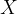$X$.$X$ will be termed a random variable of this experiment and it can take one of these possible values: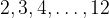$2,3,4,\ldots ,12$.Consider another random experiment wherein we toss a coin a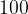$100$ times and we are interested in the number of Heads obtained, which is again a random variable of this experiment, with one of these possible values: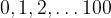$0,1,2,\ldots 100$
Of course, an experiment can have many random variables associated with it. For example, for the coin tossing experiment above, we can have so many possible random variables.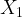${X_1}$ : No. of tails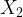${X_2}$ : No. of Heads$-$ No. of Tails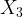${X_3}$ : The no. of tosses in the largest consecutive sequence of Heads$\vdots$ etc
Thus, we see that a random variable is a way of assigning to each outcome of the experiment, a single real number, which will vary with different outcomes of the experiment. So far, so good.
Now, we will try to understand what the probability distribution of a random variable means.
Consider once again the random experiment of rolling two dice and observing the sum of the numbers on the two dice, which we denoted by$X$$X$ can take a multitude of values in the following ways:$X$ Outcome(s) which gives this$X$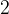$2$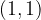$(1,1)$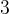$3$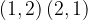$(1,2)\,(2,1)$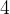$4$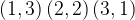$(1,3)\,(2,2)\,(3,1)$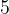$5$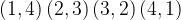$(1,4)\,(2,3)\,(3,2)\,(4,1)$$6$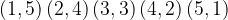$(1,5)\,(2,4)\,(3,3)\,(4,2)\,(5,1)$$7$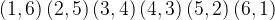$(1,6)\,(2,5)\,(3,4)\,(4,3)\,(5,2)\,(6,1)$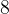$8$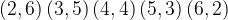$(2,6)\,(3,5)\,(4,4)\,(5,3)\,(6,2)$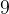$9$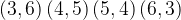$(3,6)\,(4,5)\,(5,4)\,(6,3)$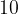$10$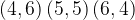$(4,6)\,(5,5)\,(6,4)$$11$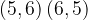$(5,6)\,(6,5)$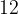$12$$(6,6)$
Each value of$X$ has a certain probability of being obtained. For example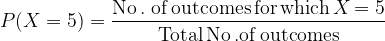$P(X = 5) = \dfrac{{{\rm{No}}\,{\rm{.}}\;{\rm{of\, outcomes \,for \,which\, }}X\,{\rm{ = 5}}}}{{{\rm{Total\, No\,}}{\rm{. of\, outcomes}}}}$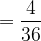$= \dfrac{4}{{36}}$$= \dfrac{1}{9}$
Let us plot the probabilities for each value of$X$:This table gives us what is known as the probability distribution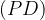$(PD)$ of$X$, that is, it is a description of how the “probability is distributed” across different values of the random variable. In simple words, the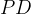$PD$ of any random variable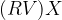$(RV) X$ tells us how probable each value of the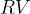$RV$ is.
The sum of the various probabilities in a$PD$ must be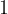$1$, as should be obvious. This fact you are urged to confirm for the last table.
Let us write down another$PD$ as an example.